# Two numbers

We have two numbers. Their sum is 140. One-fifth of the first number is equal to half the second number. Determine those unknown numbers.

x =  100
y =  40

### Step-by-step explanation:

x+y=140
x/5 = y/2

x+y = 140
2x-5y = 0

x = 100
y = 40

Our linear equations calculator calculates it.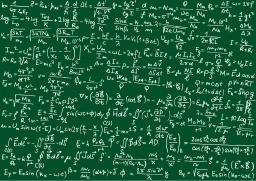Did you find an error or inaccuracy? Feel free to write us. Thank you!Math student
I just took x as 40 and y as 100 and it says I'm wrong :(Dr Math
Please read again: One-fifth of the first number is equal to half the second .... 40/5 is not equal 100/2Tips to related online calculators
Do you have a system of equations and looking for calculator system of linear equations?

## Related math problems and questions:

• Land areas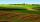Two land areas are 244 m2. The first parcel is 40 m2 less than twice the second one. What has the acreage of each parcel?
• Two numbersThe sum of the two numbers is 1. Find both numbers if you know that half of the first is equal to one-seventh of the second number.
• Two unknown numbersOne number is 2 less than a second number. Twice the second number is 14 less than 5 times the first. Find the two numbers.
• 144 stepsTo get around the garden we have to take 144 steps. 8 steps less in width than in length. One step is 5 decimetres long. What are the dimensions of the garden? What is its perimeter and its area?
• RemaindersIt is given a set of numbers { 170; 244; 299; 333; 351; 391; 423; 644 }. Divide this numbers by number 66 and determine set of remainders. As result write sum of this remainders.
• Two numbersThe sum of two numbers is 1. Identify this two numbers if you know that the half of first is equal to the third of second number.
• Ratio of two unknown numbersTwo numbers are given. Their sum is 30. We calculate one-sixth of a larger number and add to both numbers. So we get new numbers whose ratio is 5:7. Which two numbers were given?
• Four numbersThe first number is 50% second, the second number is 40% third, the third number is 20% of the fourth. The sum is 396. What are the numbers?
• Reminder and quotientThere are given numbers A = 135, B = 315. Find the smallest natural number R greater than 1 so that the proportions R:A, R:B are with the remainder 1.
• QuizQ2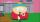The square of the first number is equal to three-fifths of the second number. Determine both numbers if you know that the second number is 5 times greater than the first, and neither of numbers is not equal to zero.
• One-thirdA one-third of unknown number is equal to five times as great as the difference of the same unknown number and number 28. Determine the unknown number.
• Probability - tickets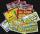What is the probability when you have 25 tickets in 5000 that you not wins the first (one) prize?
• The coil 2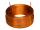The coil of a moving coil voltmeter is 40 mm long and 30 mm wide and has 100 turns on it. The control spring exerts a torque of 240 x 10-6 N-m when the deflection is 100 divisions on full scale. If the flux density of the magnetic field in the air gap is
• Cottages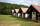The summer camp is 41 cottages. Rooms are for three and four in them. How many of the 140 campers lives of three?
• Pennies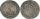800 pennies have the same value as 100 ducats. 100 pennies have the same value as 250 tolars. How many ducats has the same value as 100 tolars?
• Three unknown numbers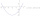The sum of of three numbers is 106. the first number is 10 more then the third. The second number is 2x the third. What are the numbers?
• Saving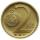Paul saves 155Kč in 46 2 Kc and 5Kc coins. How much saved 2Kc and 5Kc coins?